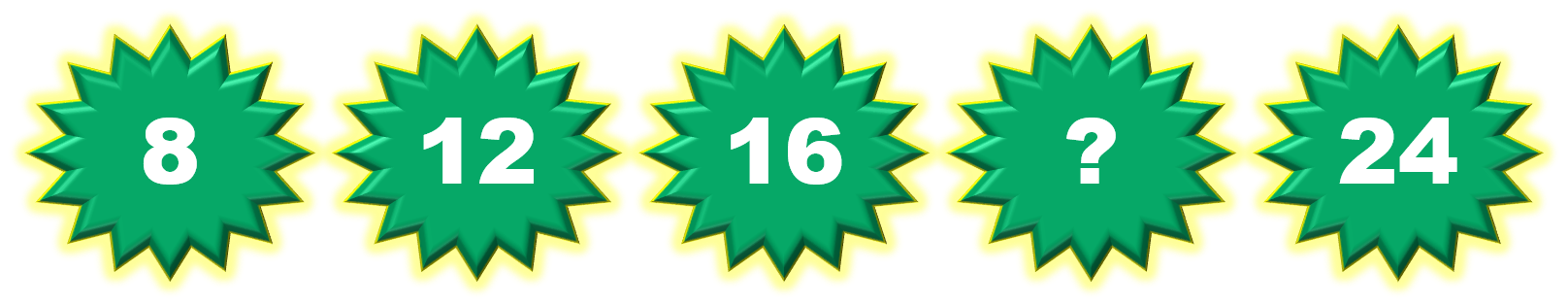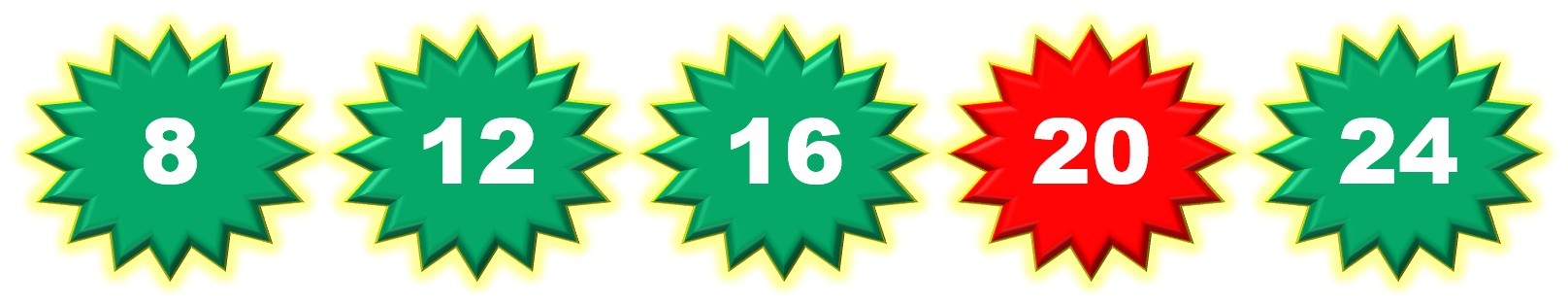1
visibility

Consider the given number pattern and identify the missing number.• A

20

• B

22

• C

18

• D

19

The correct answer is 20.

Solution:

Considering the given number pattern.Here, the numbers are increasing.
So, to find the missing number, we first find the rule.

Note that:
8 + 4 = 12
12 + 4 = 16
16 + 4 = 20
20 + 4 = 24

So, the rule is "add 4".Then, the missing number is 20.